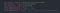# Javascript Interview and some Math solving.

01| Truthy and Falsy Values;

`function total(value){  if(value){    return "This is truty value" }else{    return "This is false value"  }}console.log(total(4))`
• This is a truthy value. Causes You using a value.
`console.log(total(0))console.log(total("0"))`
• Only “0” is the false value. Without “0” all number is a truth value. “Negative” value is a truthy value.
• If the value surrounding a Quotation mark then it is a string. And it is Truty value.
`console.log(total(false))console.log(total("false"))`
• If you using a false value then it is a false value.
• If the value surrounding a Quotation mark then it is a string. …

# Some important topics of ReactJs

01| Difference between Js and JSX

`function hello() {  return <div>Hello world!</div>;}function hello() {  return /*#__PURE__*/React.createElement("div", null, "Hello world!");}`
• When we write js then it is javaScript.
• But we write JXS then it converted js file later(Example: second function)
• When we write JXS type then the browser cannot read this type. It converts by babel and made plain simple js type.
`JXS Code:<MyButton color="blue" shadowSize={2}>  Click Me</MyButton>Compile to readable:React.createElement(  MyButton,  {color: 'blue', shadowSize: 2},  'Click Me')`
• The first code is JXS code. …

# Javascript another Ten Trips

01|Function Default Parameter:

`function add(num1, num2){num2 = num2 || 5;return num1 * num2;}const total = add(4)//output is 20function add(num1, num2 = 5){return num1 * num2;}const total = add(4)//output is 20`
• add function has two parameters. But users give one parameter.
• Then it inter the function and see the other default parameter (num2= num2 || 5).
• At last, the output is (4*5) 20.
• The second function is similar to the first function. Now I am using the default value as using with parameter.

02| Spread Operator:

`const number1 = [1,2,3,4];const number2…`

# Javascript Ten Tips

01| Includes Methods of String and ArrayImage01: String includes method

# Includes Method for String and Array

Includes Methods for String

Here “sentence” is a string. I am using the includes method. Actually includes methods is a method that searches a particular word in the main string(Ex: sentence string, Line no : 3). It gives only Boolean value. The value is “True” or “False”

If The word matches the main string then it gives a true value(Ex: Line.No: 6) or not matches it gives a false value(Ex: Line.No:7). The main String in not changes(Ex: Line.No: 8).

Includes methods for Array: